﻿ 一类刚弹混合碰撞系统的动力学分析 Analysis on a Kind of Rigid-Elastic Hybrid Collision System

Dynamical Systems and Control
Vol.07 No.02(2018), Article ID:24726,11 pages
10.12677/DSC.2018.72014

Analysis on a Kind of Rigid-Elastic Hybrid Collision System

Yugao Huangfu, Guoying Yang

School of Mathematics and Information Science, Henan Polytechnic University, Jiaozuo Henan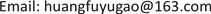Received: Mar. 29th, 2018; accepted: Apr. 23rd, 2018; published: Apr. 30th, 2018ABSTRACT

In order to study the system characteristics of a dynamic system with both rigid and elastic collisions, a new type of collisional vibration model with both rigid and elastic collisions is presented in this paper. A global Poincaré mapping method is used to analyze the conditions of one-collision- one-period and n-collisions-one-period motion vibration system. The numerical simulation simulates the system’s periodic collision movement phenomenon, and obtains the bifurcation phenomenon that occurs when the parameters change with the system. The numerical simulation method is used to study the coexistence attractors in the system.

Keywords:Piecewise Linear Collision System, Periodic Collision, Numerical Simulation1. 引言

2. 力学模型

$x 时：

$M\stackrel{¨}{x}+D\stackrel{˙}{x}+k\left(x\right)=A\mathrm{cos}\Omega t$ (1)

$x=L$ 时：

${\stackrel{˙}{x}}_{+}=-R{\stackrel{˙}{x}}_{-}$ (2)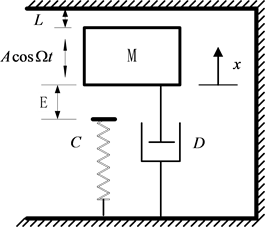Figure 1. Piecewise-linear impact dynamic system model

$\stackrel{¨}{x}+2d\stackrel{˙}{x}+k\left(x\right)=a\mathrm{cos}\Omega t,\text{\hspace{0.17em}}\text{\hspace{0.17em}}x (3)

${\stackrel{˙}{x}}_{+}=-R{\stackrel{˙}{x}}_{-},\text{\hspace{0.17em}}\text{\hspace{0.17em}}x=L$ (4)

${\stackrel{˙}{x}}_{+}=-R{\stackrel{˙}{x}}_{-}$ (5)

$\stackrel{¨}{x}+2d\stackrel{˙}{x}=a\mathrm{cos}\Omega t$ (6)

$x={C}_{1}+{C}_{2}{\text{e}}^{-2dt}+a{D}_{1}\mathrm{cos}\Omega t+a{D}_{2}\mathrm{sin}\Omega t$ (7)

$\begin{array}{l}x={C}_{1}\left({t}_{i}\right)+{C}_{2}\left({t}_{i}\right){\text{e}}^{-2d\left(t-{t}_{i}\right)}+a\left({D}_{1}\mathrm{cos}\Omega t+{D}_{2}\mathrm{sin}\Omega t\right)\\ \stackrel{˙}{x}=-2d{C}_{2}\left({t}_{i}\right){\text{e}}^{-2d\left(t-{t}_{i}\right)}-a\Omega \left({D}_{1}\mathrm{sin}\Omega t-{D}_{2}\mathrm{cos}\Omega t\right)\end{array}$ (8)

$\begin{array}{l}{C}_{1}\left({t}_{i}\right)=\frac{1}{2d}\left({y}_{i}+2d{x}_{i}-\frac{a}{\Omega }\mathrm{sin}\Omega {t}_{i}\right),\\ {C}_{2}\left({t}_{i}\right)=-\frac{1}{2d}\left[{y}_{i}+a\Omega \left({D}_{1}\mathrm{sin}\Omega {t}_{i}-{D}_{2}\mathrm{cos}\Omega {t}_{i}\right)\right],\\ {D}_{1}=\frac{-1}{{\Omega }^{2}+4{d}^{2}},{D}_{2}=\frac{2d}{\Omega \left({\Omega }^{2}+4{d}^{2}\right)}\end{array}$

$\stackrel{¨}{x}+2d\stackrel{˙}{x}+cx+e=a\mathrm{cos}\Omega t$ (9)

$x=\left({C}_{3}\mathrm{cos}\Omega t+{C}_{4}\mathrm{sin}\Omega t\right){\text{e}}^{-dt}-E+a\left({D}_{3}\mathrm{cos}\Omega t+{D}_{4}\mathrm{sin}\Omega t\right)$ (10)

$\begin{array}{l}x=\left[{C}_{3}\left({t}_{i}\right)\mathrm{cos}\omega \left(t-{t}_{i}\right)+{C}_{4}\left({t}_{i}\right)\mathrm{sin}\omega \left(t-{t}_{i}\right)\right]{\text{e}}^{-d\left(t-{t}_{i}\right)}+a\left({D}_{3}\mathrm{cos}\Omega t+{D}_{4}\mathrm{sin}\Omega t\right)\\ \stackrel{˙}{x}=\left\{\left[{C}_{4}\left({t}_{i}\right)\omega -{C}_{3}\left({t}_{i}\right)d\right]\mathrm{cos}\omega \left(t-{t}_{i}\right)-\left[{C}_{3}\left({t}_{i}\right)\omega +{C}_{4}\left({t}_{i}\right)d\right]\mathrm{sin}\omega \left(t-{t}_{i}\right)\right\}{\text{e}}^{-d\left(t-{t}_{i}\right)}\\ \text{\hspace{0.17em}}\text{\hspace{0.17em}}\text{\hspace{0.17em}}\text{\hspace{0.17em}}\text{\hspace{0.17em}}\text{\hspace{0.17em}}-a\Omega \left({D}_{3}\mathrm{sin}\Omega t-{D}_{4}\mathrm{cos}\Omega t\right)\end{array}$ (11)

$\begin{array}{l}{C}_{3}\left({t}_{i}\right)={x}_{i}+E-a\left({D}_{3}\mathrm{sin}\Omega {t}_{i}+{D}_{4}\mathrm{cos}\Omega {t}_{i}\right),\\ {C}_{4}\left({t}_{i}\right)=\frac{1}{\omega }\left[{y}_{i}+d\left({x}_{i}+E\right)-a\left(d{D}_{3}+\Omega {D}_{4}\right)\mathrm{cos}\Omega {t}_{i}-a\left(d{D}_{4}-\Omega {D}_{3}\right)\mathrm{sin}\Omega {t}_{i}\right].\end{array}$

$\omega =\sqrt{c-{d}^{2}},{D}_{3}=\frac{c-{\Omega }^{2}}{{\left(c-{\Omega }^{2}\right)}^{2}+4{d}^{2}{\Omega }^{2}},{D}_{4}=\frac{2d\Omega }{{\left(c-{\Omega }^{2}\right)}^{2}+4{d}^{2}{\Omega }^{2}}$

${\Sigma }^{+}=\left\{\left({t}_{i},{x}_{i},{y}_{i}\right)|{x}_{i}=L,{\stackrel{˙}{x}}_{i}={y}_{i}\right\}$${\Sigma }^{-}=\left\{\left({t}_{i},{x}_{i},{y}_{i}\right)|{x}_{i}=-E,{\stackrel{˙}{x}}_{i}={y}_{i}\right\}$(12)

${\Sigma }^{+}={\Sigma }_{+}^{+}\cup {\Sigma }_{-}^{+}\cup \left\{{t}_{i},L,0\right\}$${\Sigma }^{-}={\Sigma }_{+}^{-}\cup {\Sigma }_{-}^{-}\cup \left\{{t}_{i},-E,0\right\}$(13)

${\Sigma }_{+}^{+}=\left\{\left({t}_{i},{x}_{i},{y}_{i}\right)|{x}_{i}=L,{\stackrel{˙}{x}}_{i}={y}_{i}>0\right\}$${\Sigma }_{-}^{+}=\left\{\left({t}_{i},{x}_{i},{y}_{i}\right)|{x}_{i}=L,{\stackrel{˙}{x}}_{i}={y}_{i}<0\right\}$(14)

${\Sigma }_{+}^{-}=\left\{\left({t}_{i},{x}_{i},{y}_{i}\right)|{x}_{i}=-E,{\stackrel{˙}{x}}_{i}={y}_{i}>0\right\}$${\Sigma }_{-}^{-}=\left\{\left({t}_{i},{x}_{i},{y}_{i}\right)|{x}_{i}=-E,{\stackrel{˙}{x}}_{i}={y}_{i}<0\right\}$(15)

${\Sigma }_{+}^{+}$${\Sigma }_{-}^{+}$ 由点 $\left\{{t}_{i},L,0\right\}$ 连接，集 ${\Sigma }_{+}^{-}$${\Sigma }_{-}^{-}$ 由点 $\left\{{t}_{i},-E,0\right\}$ 连接。两个奇点之间的运动直接与外力有关，两个奇点之间的运动是在两个交换面之间从一端到另一端。

3. 一周期一碰和一周期n碰周期运动及其稳定性

${P}_{1}:{\Sigma }_{-}^{+}\to {\Sigma }_{-}^{-},{P}_{2}:{\Sigma }_{-}^{-}\to {\Sigma }_{+}^{-},{P}_{3}:{\Sigma }_{+}^{-}\to {\Sigma }_{+}^{+},{P}_{4}:{\Sigma }_{+}^{+}\to {\Sigma }_{-}^{+}$ (16)

$P={P}_{4}\circ {P}_{3}\circ {P}_{2}\circ {P}_{1}$ (17)

$\begin{array}{l}{P}_{1}:\left({t}_{i},L,{y}_{i}\right)\to \left({t}_{i+1},-E,{y}_{i+1}\right),\\ {P}_{2}:\left({t}_{i+1},-E,{y}_{i+1}\right)\to \left({t}_{i+2},-E,{y}_{i+2}\right),\\ {P}_{3}:\left({t}_{i+2},-E,{y}_{i+2}\right)\to \left({t}_{i+3},L,{y}_{i+3}\right),\\ {P}_{4}:\left({t}_{i+3},L,{y}_{i+3}\right)\to \left({t}_{i+4},L,{y}_{i+4}\right).\end{array}\right\}$ (18)Figure 2. MappingFigure 3. The mapping relationship

${P}_{4}\circ {P}_{3}\circ {P}_{2}\circ {P}_{1}:\left({t}_{i}L,{y}_{i}\right)\to \left({t}_{i+4},L,{y}_{i+4}\right)$ (19)

${t}_{i+4}={t}_{i}+T,{y}_{i+4}={y}_{i}$ (20)

$\begin{array}{l}E+{C}_{1}\left({t}_{i}\right)+{C}_{2}\left({t}_{i}\right){\text{e}}^{-2d\left({t}_{i+1}-{t}_{i}\right)}+a\left({D}_{1}\mathrm{cos}\Omega {t}_{i+1}+{D}_{2}\mathrm{sin}\Omega {t}_{i+1}\right)=0,\\ {y}_{i+1}+2d{C}_{2}\left({t}_{i}\right){\text{e}}^{-2d\left({t}_{i+1}-{t}_{i}\right)}+a\Omega \left({D}_{1}\mathrm{sin}\Omega {t}_{i+1}-{D}_{2}\mathrm{cos}\Omega {t}_{i+1}\right)=0.\end{array}$ (21)

$\begin{array}{l}E+\left[{C}_{3}\left({t}_{i+1}\right)\mathrm{cos}\omega \left({t}_{i+2}-{t}_{i+1}\right)+{C}_{4}\left({t}_{i+1}\right)\mathrm{sin}\omega \left({t}_{i+2}-{t}_{i+1}\right)\right]{\text{e}}^{-d\left({t}_{i+2}-{t}_{i+1}\right)}\\ \text{ }+a\left({D}_{3}\mathrm{cos}\Omega {t}_{i+2}+{D}_{4}\mathrm{sin}\Omega {t}_{i+2}\right)=0,\\ {y}_{i+2}-\left\{\left[{C}_{4}\left({t}_{i+1}\right)\omega -{C}_{3}\left({t}_{i+1}\right)d\right]\mathrm{cos}\omega \left({t}_{i+2}-{t}_{i+1}\right)\\ -\left[{C}_{3}\left({t}_{i+1}\right)\omega +{C}_{4}\left({t}_{i+1}\right)d\right]\mathrm{sin}\omega \left({t}_{i+2}-{t}_{i+1}\right)\right){\text{e}}^{-d\left({t}_{i+2}-{t}_{i+1}\right)}\\ \text{ }+a\Omega \left({D}_{3}\mathrm{sin}\Omega {t}_{i+2}-{D}_{4}\mathrm{cos}\Omega {t}_{i+2}\right)=0.\end{array}$ (22)

$\begin{array}{l}L-{C}_{1}\left({t}_{i+2}\right)-{C}_{2}\left({t}_{i+2}\right){\text{e}}^{-2d\left({t}_{i+3}-{t}_{i+2}\right)}-a\left({D}_{1}\mathrm{cos}\Omega {t}_{i+3}+{D}_{2}\mathrm{sin}\Omega {t}_{i+3}\right)=0,\\ {y}_{i+3}+2d{C}_{2}\left({t}_{i+2}\right){\text{e}}^{-2d\left({t}_{i+3}-{t}_{i+2}\right)}+a\Omega \left({D}_{1}\mathrm{sin}\Omega {t}_{i+3}-{D}_{2}\mathrm{cos}\Omega {t}_{i+3}\right)=0.\end{array}$ (23)

$\begin{array}{l}{t}_{i+4}={t}_{i+3},\\ {y}_{i+4}=-R{y}_{i+3}.\end{array}$ (24)

$DP={\left[\frac{\partial \left({t}_{i+4},{y}_{i+4}\right)}{\partial \left({t}_{i},{y}_{i}\right)}\right]}_{\left({t}_{i},{y}_{i}\right)}=\prod _{j=1}^{4}D{P}_{j}={\prod _{j=1}^{4}\left[\frac{\partial \left({t}_{i+j},{y}_{i+j}\right)}{\partial \left({t}_{i+j-1},{y}_{i+j-1}\right)}\right]}_{\left({t}_{i+j-1},{y}_{i+j-1},{t}_{i+j},{y}_{i+j}\right)}$ (25)

$P={P}_{4}{\left({P}_{5}{P}_{4}\right)}_{n-1}{P}_{3}{P}_{2}{P}_{1}$

4. 数值仿真Figure 4. One-period of n collision mappingFigure 5. Mapping diagram

5. 共存吸引子(6-1)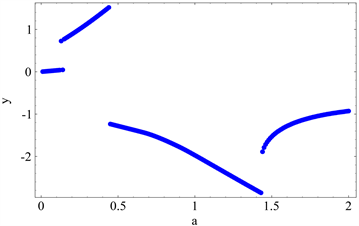(6-2)

Figure 6. System with $E=1;L=1;c=0.5;e=0.5;\Omega =0.55;d=0.05;r=0.8$ about a (1) collision frequency map (2) Poincaré mapping(7-1)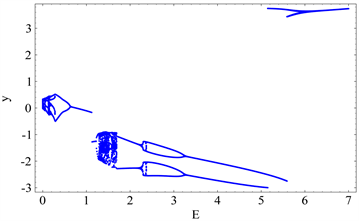(7-2)

Figure 7. Mapping of system with $L=1;c=0.9;\Omega =0.55;d=0.05;a=1;r=0.8$ about E (1) Displacement (2) Velocity Poincaré(8-1)(8-2)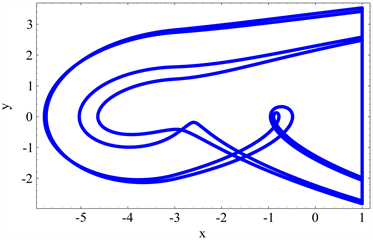(8-3)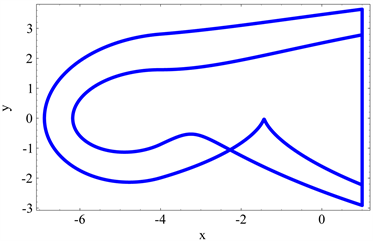(8-4)

Figure 8. Phase diagram of system with $L=1;c=0.9;\Omega =0.55;d=0.05;a=1;r=0.8$ (1) $E=1.5$ ; (2) $E=2$ ；(3) $E=3$ ; (4) $E=4$Figure 9. Coexisting attractor domain of system with parameters $E=1;L=1;c=0.5;e=0.5;\Omega =0.55;d=0.05;r=0.8;a=0.14$(10-1)(10-2)

Figure 10. Phase diagram of system with $E=1;L=1;c=0.5;e=0.5;\Omega =0.55;d=0.05;r=0.8;a=0.14$ (1) Initial point $\left(0,-0.5\right)$ (2) Initial point $\left(0.5,0.5\right)$Figure 11. Coexisting attractor domain of system with parameters $E=1;L=1;c=0.5;e=0.5;\Omega =0.55;d=0.05;r=0.8;a=1.44$(12-1)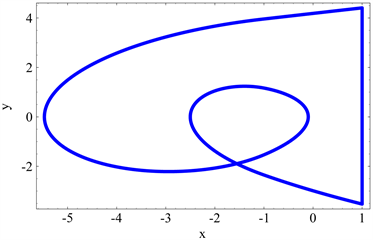(12-2)

Figure 12. Phase diagram of system with $E=1;L=1;c=0.5;e=0.5;\Omega =0.55;d=0.05;r=0.8;a=1.44$ (1) Initial point $\left(-2,0\right)$ (2) Initial point $\left(0,4\right)$

6. 结论

Analysis on a Kind of Rigid-Elastic Hybrid Collision System[J]. 动力系统与控制, 2018, 07(02): 124-134. https://doi.org/10.12677/DSC.2018.72014

1. 1. Wiggins, S. (1990) Introduction to Applied Non-linear Dynamical Systems and Chaos. Springer-Verlag, New York.

2. 2. Guckenheimer, J. and Holmes, P. (1983) Nonlinear Oscillations, Dynamical Systems and Bifurcations of Vector Fields. Springer-Verlag, New York.

3. 3. Kuznetsov, Y.A. (1998) Elements of Applied Bifurcation Theory. 2nd Edition, Springer-Verlag, New York.

4. 4. Hartog, J.P.D. and Mikina, S.J. (1932) Forced Vibrations with Non-Linear Spring Constants. Journal of Applied Mechanics, 58, 157-164.

5. 5. Shaw, S.W. and Holmes, P.J. (1983) A Periodically Forced Piecewise Linear Oscillator. Journal of Sound and Vibration, 90, 121-155.

6. 6. Nordmark, A.B. (1991) Non-Periondic Motion Caused by Grazing Incidence in an Impact Oscillator. Journal of Sound and Vibration, 145, 279-297.

7. 7. Kleczka, M., Kreuzer, E. and Schiehlen, W. (1992) Local and Global Stability of a Piecewise Linear Oscillator. Philosophical Transactions of the Royal Society A, 338, 533-546.

8. 8. Wiercigroch, M. (2000) Modeling of Dynamical System with Motion Dependent Discontinuities. Chaos, Solitons & Fractals, 11, 2429-2442.

9. 9. Luo, G.W., Xie, J.H. and Guo, S.H.L. (2001) Periodic Motions and Global Bifurcations of a Two-Degree-of-Freedom System with Plastic Vibro-Impact. Journal of Sound and Vibration, 240, 837-858.

10. 10. Luo, A.C.J. (2002) An Unsymmetrical Motion in a Horizontal Impact Oscillator. Journal of Vibration and Acoustics, 124, 420-426.

11. 11. Luo, A.C.J. and Menon, S. (2004) Global Chaos in a Periodically Forced, Linear System with a Dead-Zone Restoring Force. Chaos, Solitons and Fractals, 19, 1189-1199.
https://doi.org/10.1016/S0960-0779(03)00308-4

12. 12. Luo, A.C.J. (2005) The Mapping Dynamics of Periodic Motions for a Three-Piecewise Linear System under a Periodic Excitation. Journal of Sound and Vibration, 283, 723-748.
https://doi.org/10.1016/j.jsv.2004.05.023